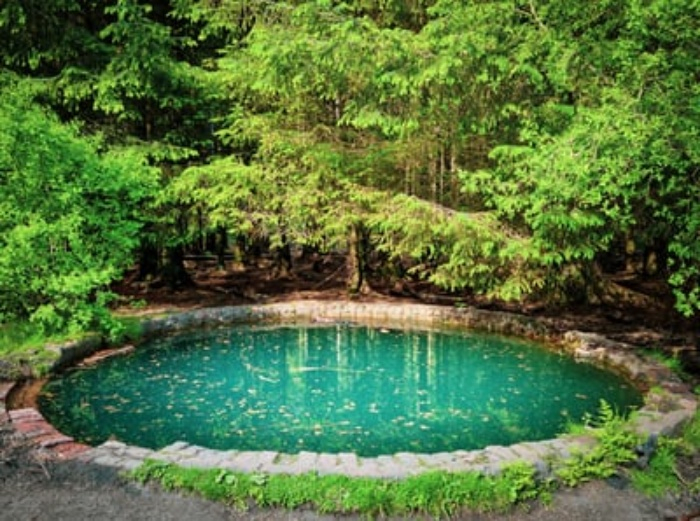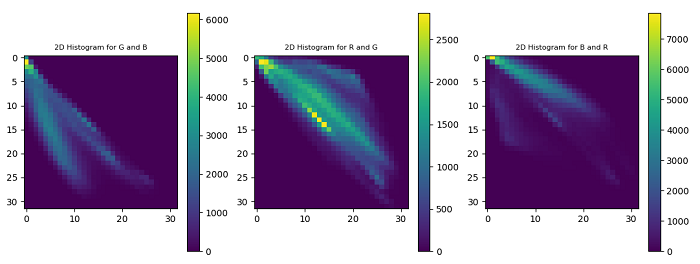# How to compute and plot 2D histograms of an image in OpenCV Python?

We can apply the cv2.calcHist() function to compute a 2D histogram of an image. The color image has three channels- Red, Green and Blue. We can compute the 2D histograms for two color channels at a time. So we have three combinations of the color channels taking two at a time- Red & Green (or Green & Red), Green & Blue (or Blue & Green) and Blue & Red (or Red & Blue).

## Steps

To compute and plot 2D histograms of an input image, one could follow the steps given below −

• Import required libraries OpenCV and matplotlib. Make sure you have already installed them.

• Read the input image using cv2.imread() method. Specify the full path of the image.

• Split the input image into the respective channels Blue, Green and Red using cv2.split() function.

blue, green, red = cv2.split(img)

• Compute the 2D color histogram for the two color channels at a time. Compute the 2D histograms for all three combinations of three color channels. To compute the 2D histogram for green and blue channels we apply below code snippet.

hist = cv2.calcHist([green, blue], [0, 1], None, [32, 32],[0, 256, 0, 256])

• Plot the above computed 2D histograms.

Let's look at some examples for a clear understanding about the question.

We use the following image as the Input File in the examples below.## Example

In this Python program, we compute and plot 2D histograms for three combinations (blue & green, green & red and red & blue) colors of the input image.

# import required libraries
import cv2
from matplotlib import pyplot as plt

# split the image into the respective channels Blue, Green and Red
blue, green, red = cv2.split(img)

# 2D color histogram for the

# green and blue channels
plt.subplot(131)
hist1 = cv2.calcHist([green, blue], [0, 1], None, [32, 32],[0, 256, 0, 256])
p = plt.imshow(hist1, interpolation="nearest")
plt.title("2D Histogram for G and B", fontsize=8)
plt.colorbar(p)

# 2D color histogram for the red and green channels
plt.subplot(132)
hist2 = cv2.calcHist([red, green], [0, 1], None, [32, 32],[0, 256, 0, 256])
p = plt.imshow(hist2, interpolation="nearest")
plt.title("2D Histogram for R and G", fontsize=8)
plt.colorbar(p)

# 2D color histogram for the blue and red channels
plt.subplot(133)
hist3 = cv2.calcHist([blue, red], [0, 1], None, [32, 32],[0, 256, 0, 256])
p = plt.imshow(hist3, interpolation="nearest")
plt.title("2D Histogram for B and R", fontsize=8)
plt.colorbar(p)
plt.show()


When you run the above program, it will produce the following output window showing the 2D histograms of the input image.Updated on: 02-Dec-2022

509 Views